EDit Software
 numberEDnumbering softwareORDER SUPPORT FREE DOWNLOAD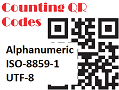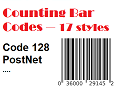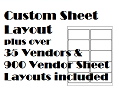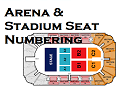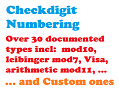# Numbering Types

numberED provides a rich selection of numbering options. A short description of each of them is explained below.

Each of these options is laid out on your design form in numberED by visually dragging / rotating a block containing a number format string to its desired location. The number format string is a set of capital Xs that indicate the desired length of the number (ex. XXXXX - a 5 character number). You can select each X to set its color, font, fontsize (thereby setting the characteristics of each corresponding digit of the number to print at that character).The X string example above may not be your choice, but serves to show how a number you might print on a form is formatted. You may just use simple single font, size, and color. Here the first two Xs were reformatted to 'Comic Sans 24pt Bold Italic Red', the middle X is 'Arial 30pt Blue', while the last two Xs are 'Comic Sans 18pt Bold Italic Black'. When the number 20466 is printed it will look as the example shows.

You may place as many numbers on your form as will fit. Just create additional number format strings.

Normal (Sequential) Numbering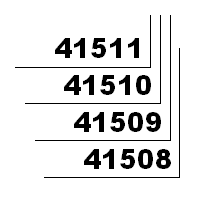Sequential numbering is counting forward or backward by 1. numberED provides this capability and allows forward or backward counting by any other value as well. (see Skip Numbering)

Bates Numbering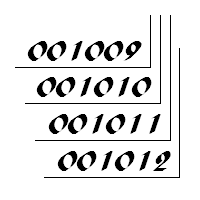Bates numbering is a six digit sequential number. numberED uses its normal (sequential) numbering capability to implement Bates numbering. You would use a six character X string. Because Bates numbering uses leading zeros when the numbers are less than 100000, you tell numberED to create leading zeros by making the first of the six Xs a zero (ex. 0XXXXX).

Book & Page NumberingIf you have a job that requires a series of books, sequentially numbered, and within each book there is a set of pages, sequentially numbered, then the Book & Page option is your chioce. Order pads is an example of such a book. The example on the left shows one book sequentially numbered from 1 to 50. The next book would be numbered 5214 and contain pages 1 to 50, ...

Checkdigit Numbering (Mod Numbering)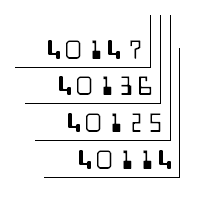Checkdigit numbering is found on routing and account numbers on checks, credit card numbers, ... Normally one of the digits in that number, the checkdigit, is the result of a formula which uses the other number digits as data. That digit is normally placed at the left or right end of the other digits. You may encounter checkdigit number terms like 'mod 10', 'mod 11', ... numberED will handle those types of checkdigit formulae and many others. numberED will properly create counting numbers, apply the select checkdigit formula, affix the checkdigit (right or left), and then print the resultant number according to the formatting indicated by the X string. The example on the left shows a four digit number using 'mod 10' to determine the checkdigit which is placed on the right end of the four digits. MICR numbering uses checkdigits. numberED's checkdigit numbering can create the digits of an MICR number. MICR fonts are available from other vendors on the Web. Be sure to use a truetype version of an MICR font for these numbers. Checkout the numberED manual section on creating checkdigit numbers.

Repeat Numbering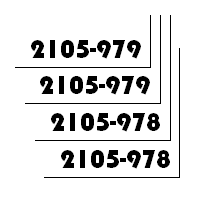numberED provides repeat numbering by allowing you to choose how many duplicate sheets are to print with the same number(s). Once those sheets print, the next number(s) are assigned to the following sheet. The example on the left shows a repeat of 2. The numbers being repeated can be any of the types numberED produces. This example is using separated numbering.

Skip Numbering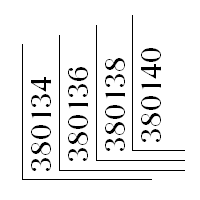With numberED you can select any value that creates a skip of that size between consecutive numbers in your print order. The example on the left shows a series of numbers skipping by 2s. These numbers have been rotated 90 degrees to read from the right of the form.

Separated NumberingThe normal use of numbers has the digits of the numbers butting against each other (ex. 23045) with no other intervening characters. Separated numbering allows you to place non-digit character(s) multiple places within the set of number digits. All keyboard characters are usable. You first make a small change to the normal X string formatting, adding carets (^) around the string. Then you add in the characters you want to separate the number digits with. The top example on the left would have an X string formatted something like '^XXac^' (the apostrophes (') are not part of the X string). The lower example would have an X string of '^X-XX-XX^'. (The carets are part of the X string and are considered to be an 'X'.) Some interesting numbering can be created (see Tiered numbering).

Tiered NumberingTiered numbers have their digits stacked above each other. Tiered numbering is a use of Separated numbering you might not notice. Remember you can use any keyboard character to separate number digits in a separated number. The ENTER key (sometimes called a carriage return or end of paragraph) is used to create a tiered number. Essentially you create a line ending with an ENTER key for each digit in the tiered number. The example on the left is a sequence of four digit tiered numbers. To create the example you use an X string formatted as a separated number and insert ENTER keys between each X of the X string '^eXeXe^' (the 'e' here represents the ENTER key). (The carets are part of the X string and are considered to be an 'X'.)